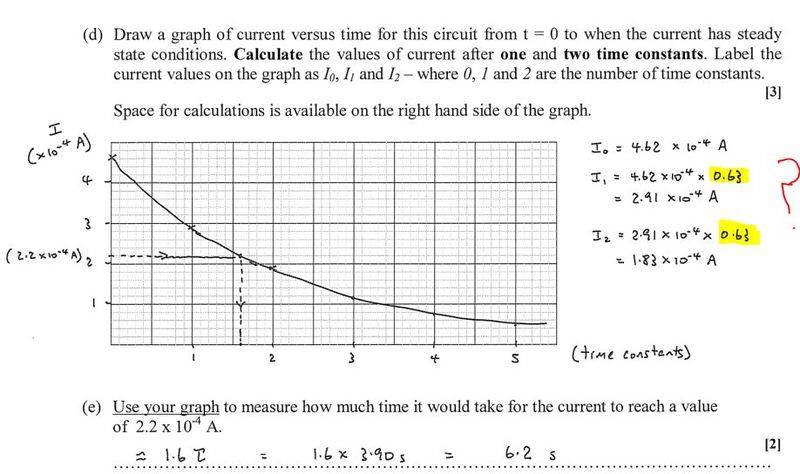# Current after (1) time constant in RC circuits

## Homework StatementMaximum current:
I max = 4.62 * 10^-4 A

Time constant of circuit:
T = 3.9 seconds

T= RC

## The Attempt at a Solution

I'm trying to find the current after 1 time constant. Seems simple enough, the current changes by 63%. So (Imax)-(Imax * 0.63) or basically I max * 0.37

But the teacher just took I max * 0.63 !!! I thought it CHANGES by 63% not simply 63% of the original value!!

I looked it up and all websites agree that the current after 1 time constant will be 0.37 of it's maximum value, hence a change of 63%!
http://www.electronics-tutorials.ws/rc/rc_1.html

$$I(t) = I_o e^{-t/\tau}$$
You're interested in the value of I(t) for various times that are multiples of $\tau$. Easy-peasy. Just divide Io by e1, e2, etc.. As you suspect, after the first time constant period the value drops to 36.8% of its initial value (1/e).